Most Affordable JEE | NEET | 8,9,10 Preparation by Kota's Top IITian Doctor Faculties

# Class 10 Notes Magnetic Effects of Electric Current

Class 10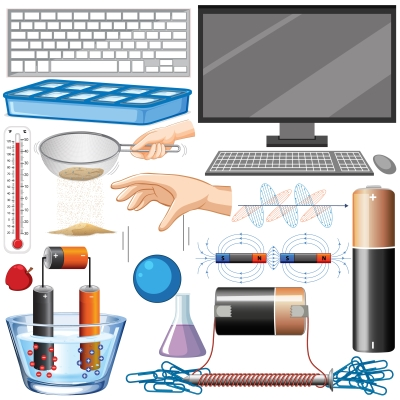`

In this article, we will explore the fascinating topic of Class 10 Notes Magnetic Effects of Electric Current, with a focus on Class 10 level. We will delve into the principles and laws governing the behavior of electric currents in the presence of magnetic fields, and how these effects have important practical applications in our daily lives. Through clear and concise notes, we aim to provide a comprehensive understanding of this topic and help students achieve mastery in this subject. So, join us on this journey to discover the magnetic world of electric currents!

Magnet -Class 10 Notes Magnetic Effects of Electric Current

Class 10 Notes Magnetic Effects of Electric Current- Magnetic field and magnetic field lines, Magnetic field due to a current carrying conductor, Right hand thumb rule, Magnetic field due to current through a circular loop. Magnetic field due to current in a solenoid.
Magnet is an object that attracts objects made of iron, cobalt and nickle. Magnet comes to rest in North – South direction, when suspended freely.

Use of Magnets: Magnets are used

• in refrigerators.
• in radio and stereo speakers.
• in audio and video cassette players.
• in children’s toys and;
• on hard discs and floppies of computers.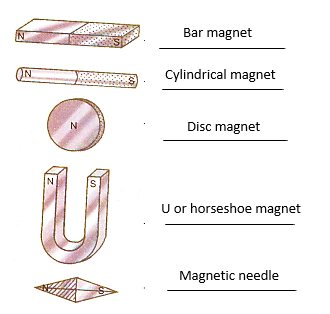Properties of Magnet

• A free suspended magnet always points towards the north and south direction.
• The pole of a magnet which points toward north direction is called north pole or north-seeking.
• The pole of a magnet which points toward south direction is called south pole or south seeking.
• Like poles of magnets repel each other while unlike poles of magnets attract each other.

Magnetic field: The area around a magnet where a magnetic force is experienced is called the magnetic field. It is a quantity that has both direction and magnitude, (i.e., Vector quantity).

Magnetic field and field lines: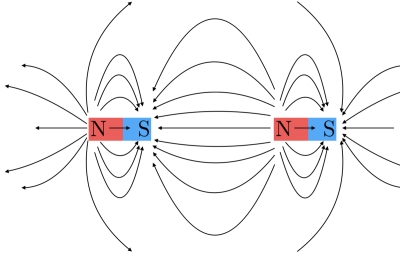The influence of force surrounding a magnet is called magnetic field. In the magnetic field, the force exerted by a magnet can be detected using a compass or any other magnet.
The magnetic field is represented by magnetic field lines.

The imaginary lines of magnetic field around a magnet are called field line or field line of magnet. When iron fillings are allowed to settle around a bar magnet, they get arranged in a pattern which mimicks the magnetic field lines. Field line of a magnet can also be detected using a compass. Magnetic field is a vector quantity, i.e. it has both direction and magnitude.

Direction of field line: Outside the magnet, the direction of magnetic field line is taken from North pole to South Pole. Inside the magnet, the direction of magnetic field line is taken from South pole to North pole.

Strength of magnetic field -Class 10 Notes Magnetic Effects of Electric Current

Class 10 Notes Magnetic Effects of Electric Current -The closeness of field lines shows the relative strength of magnetic field, i.e. closer lines show stronger magnetic field and vice – versa. Crowded field lines near the poles of magnet show more strength.

Properties of magnetic field lines
(i) They do not intersect each other.
(ii) It is taken by convention that magnetic field lines emerge from North pole and merge at the South pole. Inside the magnet, their direction is from South pole to North pole. Therefore magnetic field lines are closed curves.

Magnetic field lines due to current a current carrying straight conductor
A current carrying straight conductor has magnetic field in the form of concentric circles, around it. Magnetic field of current carrying straight conductor can be shown by magnetic field lines.
The direction of magnetic field through a current carrying conductor depends upon the direction of flow electric current.

Let a current carrying conductor be suspended vertically and the electric current is flowing from south to north. In this case, the direction of magnetic field will be anticlockwise. If the current is flowing from north to south, the direction of magnetic field will be clockwise.
The direction of magnetic field, in relation to direction of electric
current through a straight conductor can be depicted by using the Right Hand Thumb Rule. It is also known as Maxwell’s Corkscrew Rule.

Right-Hand Thumb Rule: If a current carrying conductor is held by right hand, keeping the thumb straight and if the direction of electric current is in the direction of thumb, then the direction of wrapping of other fingers will show the direction of magnetic field.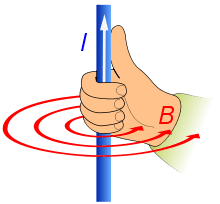Maxwell’s Corkscrew rule: As per Maxwell’s Corkscrew Rule, if the direction of forward movement of screw shows the direction of the current, then the direction of rotation of screw shows the direction of magnetic field.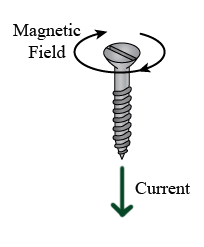Properties of magnetic field

• The magnitude of magnetic field increases with increase in electric current and decreases with decrease in electric current.
• The magnitude of magnetic field produced by electric current decreases with increase in distance and vice – versa. The size of concentric circles of magnetic field lines increases with distance from the conductor, which shows that magnetic field decreases with distance.
• Magnetic field lines are always parallel to each other.
• No two field lines cross each other.

Magnetic field lines due to a current through a circular loop
In case of a circular current carrying conductor, the magnetic field is produced in the same manner as it is in case of a straight current carrying conductor.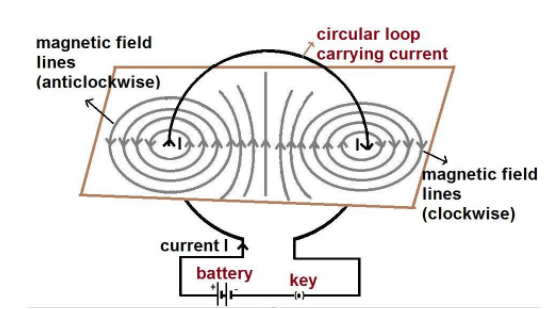In case of a circular current carrying conductor, the magnetic field lines would be in the form of iron concentric circles around every part of the FllmSs periphery of the conductor. Since, magnetic field lines tend to remain closer when near to the conductor, so the magnetic field would be stronger near the periphery of the loop. On the other hand, the magnetic field lines would be distant from each other when we move towards the centre of the current carrying loop. Finally, at the centre, the arcs of big circles would appear as a straight line.

The direction of the magnetic field can be identified using Right Hand Thumb’s Rule. Let us assume that the current is moving in anti-clockwise direction in the loop. In that case, the magnetic field would be in clockwise direction, at the top of the loop. Moreover, it would be in an anti-clockwise direction at the bottom of the loop.

Clock Face Rule: A current carrying loop works like a disc magnet. The polarity of this magnet can be easily understood with the help of Clock Face Rule. If the current is flowing in anti – clockwise direction, then the face of the loop shows north pole. On the other hand, if the current is flowing in clockwise direction, then the face of the loop shows south pole.

Magnetic field and number of turns of coil: Magnitude of magnetic field gets summed up with increase in the number of turns of coil. If there are ‘n’ turns of coil, magnitude of magnetic field will be ‘n’ times of magnetic field in case of a single turn of coil.

The strength of the magnetic field at the centre of the loop(coil) depends on :
(i) The radius of the coil: The strength of the magnetic field is inversely proportional to the radius of the coil. If the radius increases, the magnetic strength at the centre decreases
(ii) The number of turns in the coil : As the number of turns in the coil increase, the magnetic strength at the centre increases, because the current in each circular turn is having the same direction, thus, the field due to each turn adds up.
(iii) The strength of the current flowing in the coil: As the strength of the current increases, the strength of three magnetic fields also increases.

Magnetic field due to a current in a Solenoid: Solenoid is the coil with many circular turns of insulated copper wire wrapped closely in the shape of a cylinder. A current carrying solenoid produces similar pattern of magnetic field as a bar magnet. One end of solenoid behaves as the north pole and another end behaves as the south pole.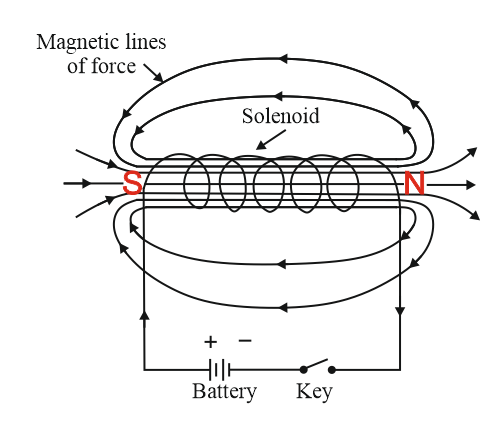Magnetic field lines are parallel inside the solenoid, similar to a bar magnet, which shows that magnetic field is same at all points inside the solenoid.
Magnetic field produced by a solenoid is similar to a bar magnet.
The strength of magnetic field is proportional to the number of turns and magnitude of current.
By producing a strong magnetic field inside the solenoid, magnetic materials can be magnetized. Magnet formed by producing magnetic field inside a solenoid is called electromagnet.

Electromagnet, Fleming’s Left-Hand Rule, Electric motor, Electromagnetic induction, Fleming’s right hand rule, Electric generator and domestic electic circuits.
Electromagnet: An electromagnet consists of a long coil of insulated copper wire wrapped on a soft iron.
Magnet formed by producing magnetic field inside a solenoid is called electromagnet.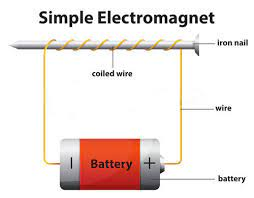Force on a current carrying conductor in a magnetic field: A current carrying conductor exerts a force when a magnet is placed in its vicinity. Similarly, a magnet also exerts equal and opposite force on the current carrying conductor. This was suggested by Marie Ampere, a French Physicist and considered as founder of science of electromagnetism.

The direction of force over the conductor gets reversed with the change in direction of flow of electric current. It is observed that the magnitude of force is highest when the direction of current is at right angles to the magnetic field.

Fleming’s Left-Hand Rule: If the direction of electric current is perpendicular to the magnetic field, the direction of force is also perpendicular to both of them. The Fleming’s Left Hand Rule states that if the left hand is stretched in a way that the index finger, the middle finger and the thumb are in mutually perpendicular directions, then the index finger and middle finger of a stretched left hand show the direction of magnetic field and direction of electric current respectively and the thumb shows the direction of motion or force acting on the conductor.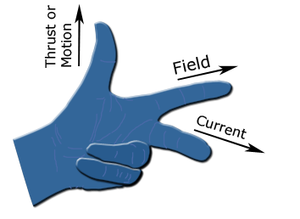The directions of electric current, magnetic field and force are similar to three mutually perpendicular axes, i.e. x, y, and z-axes.
Many devices, such as electric motor, electric generator, loudspeaker, etc. work on Fleming’s Left Hand Rule.

Electric motor: A device that converts electrical energy to mechanical energy. It is of two types : AC and DC Motor.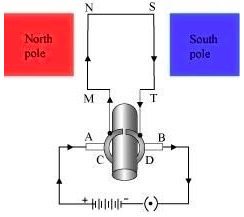Electrical energy is converted into mechanical energy by using and electric motor. Electric motor works on the basis of rule suggested by Marie Ampere and Fleming’s Left Hand Rule.

Principle of Electric Motor: When a rectangular coil is placed in a magnetic field and a current is passed through it, force acts on the coil, which rotates it continuously. With the rotation of the coil, the shaft attached to it also rotates.

Construction: It consists of the following parts :

• Armature: It is a rectangular coil (ABCD) which is suspended between the two poles of a magnetic field.
The electric supply to the coil is connected with a commutator.
• Commutator or Split – ring: Commutator is a device which reverses the direction of flow of electric current through a circuit. It is two halves of the same metallic ring.
• Magnet: Magnetic field is supplied bv a permanent magnet NS.
• Sliding contacts or Brushes Q which are fixed.
• Battery: These are consists of few cells.

Working: When an electric current is supplied to the coil of the electric motor, it gets deflected because of magnetic field. As it reaches the halfway, the split ring which acts as commutator reverses the direction of flow of electric current. Reversal of direction of the current, reverses the direction of forces acting on the coil. The change in direction of force pushes the coil, and it moves another half turn. Thus, the coil completes one rotation around the axle. Continuation of this process keeps the motor in rotation.

In commercial motor, electromagnet instead of permanent magnet and armature is used. Armature is a soft iron core with large number of conducting wire turns over it. Large number of turns of conducting wire enhances the magnetic field produced by armature.

Uses of motors :

• Used in electric fans.
• Used for pumping water.
• Used in various toys.

Electromagnetic Induction: Michael Faraday, an English Physicist is supposed to have studied the generation of electric current using a magnetic field and a conductor.
Electricity production as a result of magnetism (induced current) is called Electromagnetic Induction.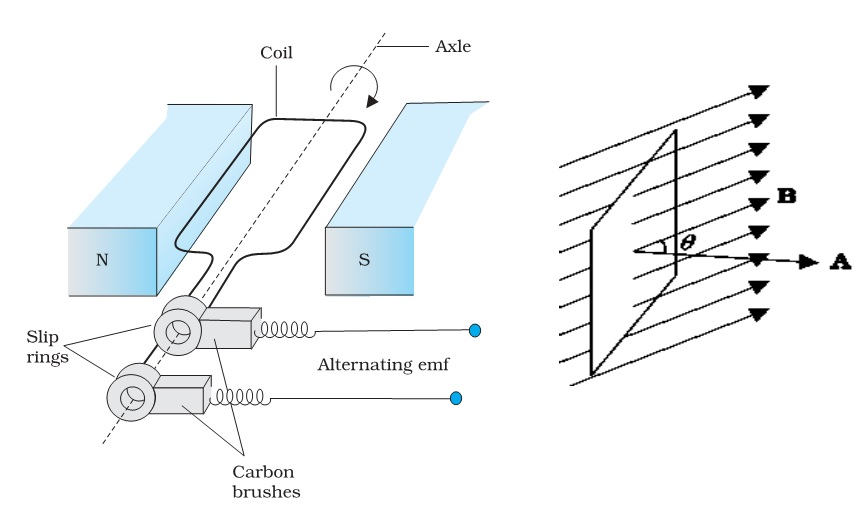When a conductor is set to move inside a magnetic field or a magnetic field is set to be changing around a conductor, electric current is induced in the conductor. This is just opposite to the exertion of force by a current carrying conductor inside a magnetic field. In other words, when a conductor is brought in relative motion vis – a – vis a magnetic field, a potential difference is induced in it. This is known as electromagnetic induction.

Fleming’s Right-Hand Rule - Class 10 Notes Magnetic Effects of Electric Current

Class 10 Notes Magnetic Effects of Electric Current- Electromagnetic induction can be explained with the help of Fleming’s Right Hand Rule. If the right hand is structured in a way that the index (fore ginger) finger, middle finger and thumb are in mutually perpendicular directions, then the thumb shows direction of induced current in the conductor, in conductor The directions of movement of conductor, magnetic field and induced current can be compared to three mutually perpendicular axes, i.e. x, y and z axes.The mutually perpendicular directions also point to an important fact that when the magnetic field and movement of conductor are perpendicular, the magnitude of induced current would be maximum.
Electromagnetic induction is used in the conversion of kinetic energy into electrical energy.

Electric Generator: A device that converts mechanical energy into electrical energy is called an electric generator.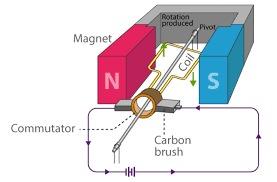Electric generators are of two types: AC generator and a DC generator. Principle of electric generator: Electric motor works on the basis of electromagnetic induction.

Construction and Working: The structure of an electric generator is similar to that of an electric motor. In case of an electric generator, a rectangular armature is placed within the magnetic field of a permanent magnet. The armature is attached to wire and is positioned in a way that it can move around an axle. When the armature moves within the magnetic field, an electric current is induced. The direction of induced current changes, when the armature crosses the halfway mark of its rotation.

Thus, the direction of current changes once in every rotation. Due to this, the electric generator usually produces alternate current, i.e. A.C. To convert an A.C generator into a D.C generator, a split ring commutator is used. This helps in producing direct current.
Electrical generator is used to convert mechanical energy into electrical energy.

A.C and D.C Current
A.C – Alternate Current: Current in which direction is changed periodically is called Alternate Current. In India, most of the power stations generate alternate current. The direction of current changes after every 1/100 second in India, i.e. the frequency of A.C in India is 50 Hz. A.C is transmitted upto a long distance without much loss of energy is advantage of A.C over D.C

D.C – Direct Current: Current that flows in one direction only is called Direct current. Electrochemical cells produce direct current.

• Cost of generatior of A.C is much less than that of D.C.
• A.C can be easily converted to D.C.
• A.C can be controlled by the use of choke which involves less loss of power whereas, D.C can be controlled using resistances which involves high energy loss.
• AC can be transmitted over long distances without much loss of energy.
• AC machines are stout and durable and do not need much maintenance.

• AC cannot be used for the electrolysis process or showing electromagnetism as it reverses its polarity.
• AC is more dangerous than DC.

Domestic Electric Circuits: We receive electric supply through mains supported through the poles or cables. In our houses, we receive AC electric power of 220 V with a frequency of 50 Hz.
The 3 wires are as follows

• Live wire – (Red insulated, Positive)
• Neutral wire – (Black insulated, Negative)
• Earth wire – (Green insulated) for safety measure to ensure that any leakage of current to a metallic body does not give any serious shock to a user.

Short Circuit: Short-circuiting is caused by the touching of live wires and neutral wire and sudden a large current flows.
It happens due to

• damage pf insulation in power lines.
• a fault in an electrical appliance.

Overloading of an Electric Circuit: The overheating of electrical wire in any circuit due to the flow of a large current through it is called overloading of the electrical circuit.
A sudden large amount of current flows through the wire, which causes overheating of wire and may cause fire also.

Electric Fuse: It is a protective device used for protecting the circuit from short-circuiting and overloading. It is a piece of thin wire of material having a low melting point and high resistance.

• Fuse is always connected to live wire.
• Fuse is always connected in series to the electric circuit.
• Fuse is always connected to the beginning of an electric circuit.
• Fuse works on the heating effect.

Magnetic field: The area around a magnet in which other magnet feels force of attraction or repulsion is called Magnetic field.

Magnetic field lines: The closed curved imaginary lines in the magnetic field which indicate the direction of motion of north pole in the magnetic field if a magnet is free to do so.

Properties of magnetic field lines.

• Magnetic Field lines originate from the north pole of a magnet and end at its south pole.
• Magnetic Field lines are denser near the poles but rarer at other places.
• The Magnetic Field lines do not intersect one another.

Oersted’s experiment: According to this experiment “A current carrying wire creates a magnetic field around it. The direction of magnetic field depends on the direction of current in conductor.”

• Magnetic field pattern due to straight current carrying conductor are concentric circles whose center lie on the wire.
• The direction of magnetic field due to straight current carrying conductor can be determined by Right hand thumb rule.

Right hand thumb rule: According to this rule “if current carrying conductor is held in the right hand in such a way that thumb indicate the direction of current, then the curled finger indicates the direction of magnetic field lines around conductor.”

Magnetic field pattern due to current carrying loop: The Magnetic field lines are circular near the current-carrying loop. As we move away from the loop, field lines form bigger and bigger circles. At the center of the circular loop, the magnetic field lines are straight.

The solenoid is an insulated and tightly wound long circular wire having large number of turns whose radius is small in comparison to its length. Magnetic field produced by a solenoid is similar to the magnetic field produced by a bar magnet.

Current carrying solenoid is called an electromagnet.

Properties of magnetic lines of force or magnetic field lines.

• These lines originate from the north pole and end at the south pole.
• The magnetic field lines of a magnet form a continuous closed loop.
• Two magnetic lines of force do not intersect each other.
• The tangent at any point on the magnetic line gives the direction of the magnetic field at the point.

Fleming’s left hand rule: According to this rule, “if the thumb, forefinger and middle finger of the left hand are stretched perpendicular to each other and if the fore-finger gives the direction of magnetic field, middle finger gives the direction of current, then the thumb will give the direction of motion or the force acting on the current-carrying conductor.”

Principle of an electric motor: A motor works on the principle that when a rectangular coil is placed in a magnetic field and current passes through it, a force acts on the coil which rotates it continuously.
When the coil rotates, the shaft attached to it also rotates. In this way the electrical energy supplied to the motor is converted into the mechanical energy of rotation.

Principle of an electric generator: It is based on the principle of electromagnetic induction. It states that “an induced current is produced in a coil placed in a region where the magnetic field changes with time.” The direction of induced current is given by Fleming’s right-hand rule. An electric generator converts mechanical energy into electrical energy.

Electromagnetic induction: The phenomenon of setting up of an electric current or an induced e.mi. by changing the magnetic lines of force by a moving conductor is called electromagnetic induction.

Maxwell’s right hand thumb rule: The direction of the current is given by Maxwell’s right-hand thumb rule, “If the current carrying conductor is gripped with the right hand in such a way that the thumb gives the direction of the current, then the direction of the fingers gives the direction of the magnetic field produced around the conductor.

Fleming’s left-hand rule: The direction of motion of a conductor in a magnetic field is given by Fleming’s left-hand rule. According to this rule, if the thumb, forefinger and middle finger of the left hand are stretched perpendicular to each other and if fore-finger gives the direction of the magnetic field and the middle finger gives the direction of current then, the thumb will give the direction of the motion of the conductor carrying the current.

Fleming’s right-hand rule: The direction of the induced current is given by Fleming’s right-hand rule. According to this rule if the thumb, forefinger and middle finger of the right hand are stretched perpendicular to each other and if the fore-finger gives the direction of the magnetic field and the thumb gives the direction of motion, then the middle finger will give the direction of the induced current in the conductor.

### Conclusion

In conclusion, we hope that these Class 10 Notes Magnetic Effects of Electric Current have provided you with a solid foundation in this fascinating topic. By understanding the principles and laws governing the behavior of electric currents in the presence of magnetic fields, you can appreciate the important practical applications of this field, such as in motors and generators. By reviewing these notes regularly, you can ensure that you have a deep understanding of this subject and perform well in your exams. So, keep studying and exploring the magnetic world of electric currents with the help of these Class 10 Notes Magnetic Effects of Electric Current!

Download the eSaral App for complete Class 10 Video lectures, Study material, revision and much more.

Class 10 Notes Magnetic Effects of Electric Current